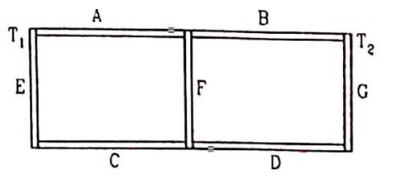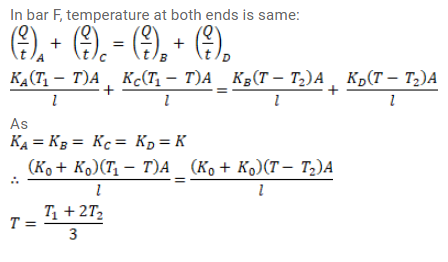# Seven rods A, B, C, D, E, F and G are joined as shown in figure.

Question:

Seven rods A, B, C, D, E, F and $\mathrm{G}$ are joined as shown in figure. All the rods have equal cross - sectional area A and the length I. The thermal conductivities of the rods are $\mathrm{K}_{\mathrm{A}}=\mathrm{K}_{\mathrm{C}}=\mathrm{K}_{0}, \mathrm{~K}_{\mathrm{B}}=\mathrm{K}_{\mathrm{D}}=2 \mathrm{~K}_{0}, \mathrm{~K}_{\mathrm{E}}=3 \mathrm{~K}_{0}, \mathrm{~K}_{\mathrm{F}}=4 \mathrm{~K}_{0}$ and $\mathrm{K}_{\mathrm{G}}=5 \mathrm{~K}_{0}$. The rod $\mathrm{E}$ is kept at a constant temperature $T_{1}$ and the rod $G$ is kept at a constant temperature $T_{2}\left(T_{2}>T_{1}\right)$ (a) Show that the rod $F$ has a uniform temperature $T=\left(T_{1}+2 T_{2}\right) / 3$. (b) Find the rate of heat flow from the source which maintains the temperature $T_{2}$.Solution: